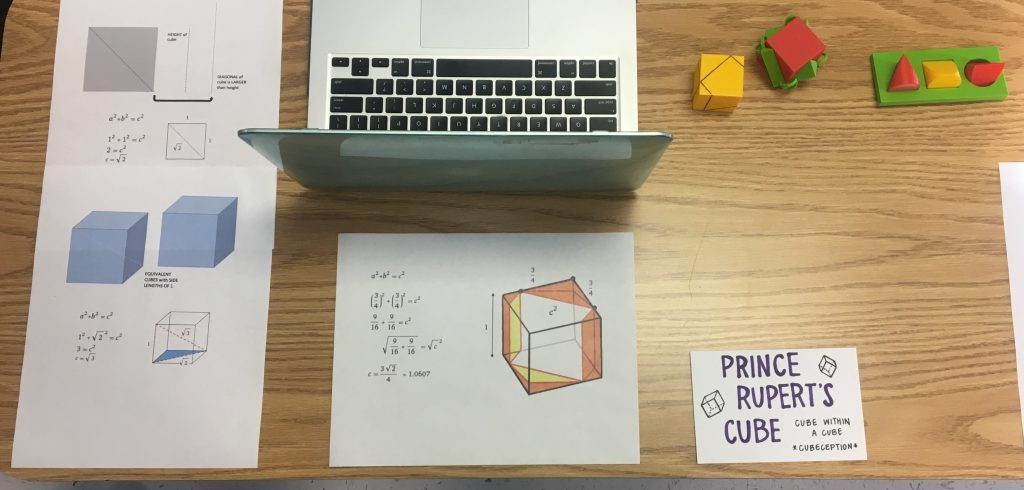# Prince Rupert’s Cube Showcase

Taylor Zabinsky
Grant Folsom

## Prince Rupert’s Cube Presentation:Our final presentation was on Prince Rupert’s Cube. Essentially, the Prince Rupert problem was proposed by Prince Rupert of the Rhine, Prince Rupert’s cube is the largest cube that can pass through a hole cut through another cube without splitting the cube into two pieces. One would assume that the only sized cube that could pass through the orginial would be one of equivalent size. What if you passed it through on the diagonal? The diagonal of a cube with the dimensions of 1 is actually larger than the side height. So yes, a larger cube would be able to pass through the orginial cube if it was put through on the diagonal. After using the pythagorean theorem to calculate the length of the diagonal, the solution to the problem is a maximally sized inner cube with sides of length 1.0606 (3 sqrt(2))/4) larger than the unit cube with side lengths of 1. You can read more about Prince Rupert’s Cube here.

We think our final presentation was a success. We had numerous people ask us many questions about our project, about how the design was constructed and how this could be applied to real life situations.We also had to oppportunity to walk around and ask other groups about their projects. One that we particulary enjoyed was the Borromean Rings. They explained their project very well and amazed us with how the rings actually work, come together and pull apart. In addition, the ribbon braid was a neat visual.